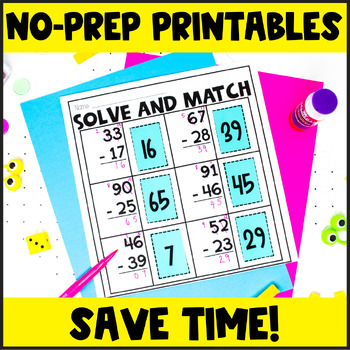EASEL BY TPT

Total:
\$0.00# 2 Digit Subtraction with Regrouping2nd - 3rd
Subjects
Standards
Resource Type
Formats Included
• Zip
•Google Apps™
Pages
65 pages
\$6.75
\$6.75The Teacher-Author indicated this resource includes assets from Google Workspace (e.g. docs, slides, etc.).

#### Also included in

1. Looking for an all-in-one solution for activities to teach Double-digit addition and subtraction? This 2-digit resource with regrouping bundle features math centers, games, step-by-step lessons, posters, crafts, and more! I've also included equations without regrouping to help differentiate!This Dou
\$17.14
\$20.05
Save \$2.91

### Description

Double digit subtraction with regrouping doesn't have to be a struggle! These double digit subtraction with regrouping posters, printables, flapbooks, activities, and worksheets will save you time as a teacher while also providing your students with the practice they need!

Whether you are just starting to teach subtraction with regrouping, or your students are reviewing, this resource has lots of activities your students can do!

Double Digit Subtraction With Regrouping Includes:

• Teaching Tips
• 4 Subtraction Posters to teach subtraction rhymes (in color and black and white)
• Posters for students
• Ladybug Activity with all templates included to review checking subtraction problems.
• Elephant Activity with all templates included to review checking subtraction problems.
• "I Spy" Subtraction with regrouping activity and cards
• Subtraction with regrouping word problems (digital and printable)
• Subtraction and Addition Key Words Sort
• Subtraction with regrouping writing prompt
• Student Workmat
• Place Value Mats
• Subtraction with regrouping worksheet
• Subtraction with regrouping activity with even/odd sort (digital and printable)
• Subtraction with regrouping flapbook- Students solve problems and sort based on the subtraction rhyme
• Subtraction and Addition pocket book- Students solve subtraction and addition with and without regrouping problems and sort into the correct pocket
• Solve and Match (2 printables): Students solve 6 subtraction problems and match the differences to each problem. (digital and printable)
• Solve and Match by Even/Odd (2 printables): Students solve 6 subtraction problems and identify whether the difference is even or odd (digital and printable)
• 4 Flapbooks: 2 Solve and Show your work, 1 sort by even/odd, 1 sort by regroup/not regroup (digital and printable)

UPDATE: 8 of the activities are now DIGITAL! These activities can be accessed through a link to Google Slides and Seesaw. They are preloaded for you to access easily. There is a how-to video included for you to view.

Looking for more regrouping activities? Check out below!

Two Digit Subtraction With Regrouping

Two Digit Regrouping Centers

Three Digit Subtraction with Regrouping

Three Digit Addition and Subtraction with Regrouping Centers

Magic of Math 2nd Grade Regrouping

Magic of Math 3rd Grade Regrouping

2 Digit Regrouping Bundle

3 Digit Regrouping Bundle

Total Pages
65 pages
Not Included
Teaching Duration
N/A
Report this Resource to TpT
Reported resources will be reviewed by our team. Report this resource to let us know if this resource violates TpT’s content guidelines.

### Standards

to see state-specific standards (only available in the US).
Use addition and subtraction within 100 to solve one- and two-step word problems involving situations of adding to, taking from, putting together, taking apart, and comparing, with unknowns in all positions, e.g., by using drawings and equations with a symbol for the unknown number to represent the problem.
Fluently add and subtract within 100 using strategies based on place value, properties of operations, and/or the relationship between addition and subtraction.
Add up to four two-digit numbers using strategies based on place value and properties of operations.
Add and subtract within 1000, using concrete models or drawings and strategies based on place value, properties of operations, and/or the relationship between addition and subtraction; relate the strategy to a written method. Understand that in adding or subtracting three-digit numbers, one adds or subtracts hundreds and hundreds, tens and tens, ones and ones; and sometimes it is necessary to compose or decompose tens or hundreds.